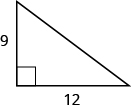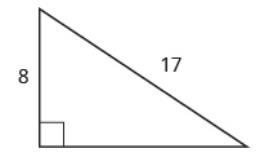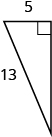$$\newcommand{\id}{\mathrm{id}}$$ $$\newcommand{\Span}{\mathrm{span}}$$ $$\newcommand{\kernel}{\mathrm{null}\,}$$ $$\newcommand{\range}{\mathrm{range}\,}$$ $$\newcommand{\RealPart}{\mathrm{Re}}$$ $$\newcommand{\ImaginaryPart}{\mathrm{Im}}$$ $$\newcommand{\Argument}{\mathrm{Arg}}$$ $$\newcommand{\norm}{\| #1 \|}$$ $$\newcommand{\inner}{\langle #1, #2 \rangle}$$ $$\newcommand{\Span}{\mathrm{span}}$$

# 9.5: Use Properties of Angles, Triangles, and the Pythagorean Theorem (Part 2)

•• Contributed by OpenStax
• Mathematics at OpenStax CNX
$$\newcommand{\vecs}{\overset { \rightharpoonup} {\mathbf{#1}} }$$ $$\newcommand{\vecd}{\overset{-\!-\!\rightharpoonup}{\vphantom{a}\smash {#1}}}$$$$\newcommand{\id}{\mathrm{id}}$$ $$\newcommand{\Span}{\mathrm{span}}$$ $$\newcommand{\kernel}{\mathrm{null}\,}$$ $$\newcommand{\range}{\mathrm{range}\,}$$ $$\newcommand{\RealPart}{\mathrm{Re}}$$ $$\newcommand{\ImaginaryPart}{\mathrm{Im}}$$ $$\newcommand{\Argument}{\mathrm{Arg}}$$ $$\newcommand{\norm}{\| #1 \|}$$ $$\newcommand{\inner}{\langle #1, #2 \rangle}$$ $$\newcommand{\Span}{\mathrm{span}}$$ $$\newcommand{\id}{\mathrm{id}}$$ $$\newcommand{\Span}{\mathrm{span}}$$ $$\newcommand{\kernel}{\mathrm{null}\,}$$ $$\newcommand{\range}{\mathrm{range}\,}$$ $$\newcommand{\RealPart}{\mathrm{Re}}$$ $$\newcommand{\ImaginaryPart}{\mathrm{Im}}$$ $$\newcommand{\Argument}{\mathrm{Arg}}$$ $$\newcommand{\norm}{\| #1 \|}$$ $$\newcommand{\inner}{\langle #1, #2 \rangle}$$ $$\newcommand{\Span}{\mathrm{span}}$$

## Use the Pythagorean Theorem

The Pythagorean Theorem is a special property of right triangles that has been used since ancient times. It is named after the Greek philosopher and mathematician Pythagoras who lived around 500 BCE.

Remember that a right triangle has a 90° angle, which we usually mark with a small square in the corner. The side of the triangle opposite the 90° angle is called the hypotenuse, and the other two sides are called the legs. See Figure 9.12.Figure 9.12 - In a right triangle, the side opposite the 90° angle is called the hypotenuse and each of the other sides is called a leg.

The Pythagorean Theorem tells how the lengths of the three sides of a right triangle relate to each other. It states that in any right triangle, the sum of the squares of the two legs equals the square of the hypotenuse.

Definition: The Pythagorean Theorem

In any right triangle ΔABC,

$$a^{2} + b^{2} = c^{2}$$

where c is the length of the hypotenuse a and b are the lengths of the legs.To solve problems that use the Pythagorean Theorem, we will need to find square roots. In Simplify and Use Square Roots we introduced the notation m and defined it in this way:

$$If\; m = n^{2}, then\; \sqrt{m} = n\; for\; n \geq 0$$

For example, we found that $$\sqrt{25}$$ is 5 because 52 = 25.

We will use this definition of square roots to solve for the length of a side in a right triangle.

Example 9.22:

Use the Pythagorean Theorem to find the length of the hypotenuse.Solution

 Step 1. Read the problem. Step 2. Identify what you are looking for. the length of the hypotenuse of the triangle Step 3. Name. Choose a variable to represent it. Let c = the length of the hypotenuseStep 4. Translate. Write the appropriate formula. Substitute. $$\begin{split} a^{2} + b^{2} &= c^{2} \\ 3^{2} + 4^{2} &= c^{2} \end{split}$$ Step 5. Solve the equation. $$\begin{split} 9 + 16 &= c^{2} \\ 25 &= c^{2} \\ \sqrt{25} &= c^{2} \\ 5 &= c \end{split}$$ Step 6. Check. $$\begin{split} 3^{2} + 4^{2} &= \textcolor{red}{5}^{2} \\ 9 + 16 &\stackrel{?}{=} 25 \\ 25 &= 25\; \checkmark \end{split}$$ Step 7. Answer the question. The length of the hypotenuse is 5.

Exercise 9.43:

Use the Pythagorean Theorem to find the length of the hypotenuse.Exercise 9.44:

Use the Pythagorean Theorem to find the length of the hypotenuse.Example 9.23:

Use the Pythagorean Theorem to find the length of the longer leg.Solution

 Step 1. Read the problem. Step 2. Identify what you are looking for. The length of the leg of the triangle Step 3. Name. Choose a variable to represent it. Let b = the leg of the triangle Label side bStep 4. Translate. Write the appropriate formula. Substitute. $$\begin{split} a^{2} + b^{2} &= c^{2} \\ 5^{2} + b^{2} &= 13^{2} \end{split}$$ Step 5. Solve the equation. $$\begin{split} 25 + b^{2} &= 169 \\ b^{2} &= 144 \\ b^{2} &= \sqrt{144} \\ b &= 12 \end{split}$$ Step 6. Check. $$\begin{split} 5^{2} + \textcolor{red}{12}^{2} &\stackrel{?}{=} 13^{2} \\ 25 + 144 &\stackrel{?}{=} 169 \\ 169 &= 169\; \checkmark \end{split}$$ Step 7. Answer the question. The length of the leg is 12.

Exercise 9.45:

Use the Pythagorean Theorem to find the length of the leg.Exercise 9.46:

Use the Pythagorean Theorem to find the length of the leg.Example 9.24:

Kelvin is building a gazebo and wants to brace each corner by placing a 10-inch wooden bracket diagonally as shown. How far below the corner should he fasten the bracket if he wants the distances from the corner to each end of the bracket to be equal? Approximate to the nearest tenth of an inch.Solution

 Step 1. Read the problem. Step 2. Identify what you are looking for. the distance from the corner that the bracket should be attached Step 3. Name. Choose a variable to represent it. Let x = the distance from the cornerStep 4. Translate. Write the appropriate formula. Substitute. $$\begin{split} a^{2} + b^{2} &= c^{2} \\ x^{2} + x^{2} &= 10^{2} \end{split}$$ Step 5. Solve the equation. Isolate the variable. Use the definition of the square root. Simplify. Approximate to the nearest tenth. $$\begin{split} 2x^{2} &= 100 \\ x^{2} &= 50 \\ x &= \sqrt{50} \\ b &\approx 7.1 \end{split}$$ Step 6. Check. $$\begin{split} a^{2} + b^{2} &= c^{2} \\ (\textcolor{red}{7.1})^{2} + (\textcolor{red}{7.1})^{2} &\stackrel{?}{\approx} 10^{2} \\ &Yes. \end{split}$$ Step 7. Answer the question. Kelvin should fasten each piece of wood approximately 7.1" from the corner.

Exercise 9.47:

John puts the base of a 13-ft ladder 5 feet from the wall of his house. How far up the wall does the ladder reach?Exercise 9.48:

Randy wants to attach a 17-ft string of lights to the top of the 15-ft mast of his sailboat. How far from the base of the mast should he attach the end of the light string?## Practice Makes Perfect

### Use the Properties of Angles

In the following exercises, find (a) the supplement and (b) the complement of the given angle.

1. 53°
2. 16°
3. 29°
4. 72°

In the following exercises, use the properties of angles to solve.

1. Find the supplement of a 135° angle.
2. Find the complement of a 38° angle.
3. Find the complement of a 27.5° angle.
4. Find the supplement of a 109.5° angle.
5. Two angles are supplementary. The larger angle is 56° more than the smaller angle. Find the measures of both angles.
6. Two angles are supplementary. The smaller angle is 36° less than the larger angle. Find the measures of both angles.
7. Two angles are complementary. The smaller angle is 34° less than the larger angle. Find the measures of both angles.
8. Two angles are complementary. The larger angle is 52° more than the smaller angle. Find the measures of both angles.

### Use the Properties of Triangles

In the following exercises, solve using properties of triangles.

1. The measures of two angles of a triangle are 26° and 98°. Find the measure of the third angle.
2. The measures of two angles of a triangle are 61° and 84°. Find the measure of the third angle.
3. The measures of two angles of a triangle are 105° and 31°. Find the measure of the third angle.
4. The measures of two angles of a triangle are 47° and 72°. Find the measure of the third angle.
5. One angle of a right triangle measures 33°. What is the measure of the other angle?
6. One angle of a right triangle measures 51°. What is the measure of the other angle?
7. One angle of a right triangle measures 22.5°. What is the measure of the other angle?
8. One angle of a right triangle measures 36.5°. What is the measure of the other angle?
9. The two smaller angles of a right triangle have equal measures. Find the measures of all three angles.
10. The measure of the smallest angle of a right triangle is 20° less than the measure of the other small angle. Find the measures of all three angles.
11. The angles in a triangle are such that the measure of one angle is twice the measure of the smallest angle, while the measure of the third angle is three times the measure of the smallest angle. Find the measures of all three angles.
12. The angles in a triangle are such that the measure of one angle is 20° more than the measure of the smallest angle, while the measure of the third angle is three times the measure of the smallest angle. Find the measures of all three angles.

### Find the Length of the Missing Side

In the following exercises, Δ ABC is similar to Δ XYZ. Find the length of the indicated side.1. side b
2. side x

On a map, San Francisco, Las Vegas, and Los Angeles form a triangle whose sides are shown in the figure below. The actual distance from Los Angeles to Las Vegas is 270 miles.1. Find the distance from Los Angeles to San Francisco.
2. Find the distance from San Francisco to Las Vegas.

### Use the Pythagorean Theorem

In the following exercises, use the Pythagorean Theorem to find the length of the hypotenuse.

1.2.3.4.### Find the Length of the Missing Side

In the following exercises, use the Pythagorean Theorem to find the length of the missing side. Round to the nearest tenth, if necessary.

1.2.3.4.5.6.7.8.In the following exercises, solve. Approximate to the nearest tenth, if necessary.

1. A 13-foot string of lights will be attached to the top of a 12-foot pole for a holiday display. How far from the base of the pole should the end of the string of lights be anchored?1. Pam wants to put a banner across her garage door to congratulate her son on his college graduation. The garage door is 12 feet high and 16 feet wide. How long should the banner be to fit the garage door?1. Chi is planning to put a path of paving stones through her flower garden. The flower garden is a square with sides of 10 feet. What will the length of the path be?1. Brian borrowed a 20-foot extension ladder to paint his house. If he sets the base of the ladder 6 feet from the house, how far up will the top of the ladder reach?## Everyday Math

1. Building a scale model Joe wants to build a doll house for his daughter. He wants the doll house to look just like his house. His house is 30 feet wide and 35 feet tall at the highest point of the roof. If the dollhouse will be 2.5 feet wide, how tall will its highest point be?
2. Measurement A city engineer plans to build a footbridge across a lake from point X to point Y, as shown in the picture below. To find the length of the footbridge, she draws a right triangle XYZ, with right angle at X. She measures the distance from X to Z, 800 feet, and from Y to Z, 1,000 feet. How long will the bridge be?## Writing Exercises

1. Write three of the properties of triangles from this section and then explain each in your own words.
2. Explain how the figure below illustrates the Pythagorean Theorem for a triangle with legs of length 3 and 4.## Self Check

(a) After completing the exercises, use this checklist to evaluate your mastery of the objectives of this section.(b) What does this checklist tell you about your mastery of this section? What steps will you take to improve?